# Date to month in excel

Sometimes, the users have a date and want to calculate the month. Excel enables several methods to its users to find the month from a date. A user has this type of requirement when he wants to calculate the total number of years, months, and dates.

In this chapter, we will help you to convert the date to month using MS Excel functions and features. Additionally, we will also calculate the years, months, and days from a date. Follow this tutorial below.

## Methods to convert date to months

Whenever a user enters a date into an Excel cell, it automatically converts the cell as a date cell by recognizing it. It means that MS Excel is very known with the year, month, and day of the date. Since Excel understands the date, the user can easily extract the month from the date.

Excel enables various ways to convert the date to months.

All are useful methods in their ways for conversion from date to weekdays/months/years.

## Extract date to month using MONTH() function

Excel MONTH() and YEAR() methods help the users to extract the month and year from a date. The date should be in a valid Excel date if the user wants to use this function. If the date is not correct, you can get the #VALUE error on using this function.

The YEAR() function will also return the #VALUE error on finding an invalid date.

Example

Step 1: We take some sample data to see the month extraction from a valid date using the MONTH() function. The data for different dates is as follows:Step 2: Click on a blank cell where the extracted result will be pasted and write the following formula for month extraction.

=MONTH(B2)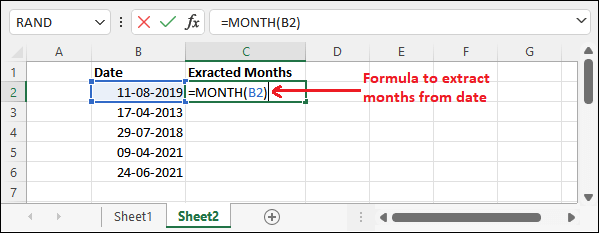Step 3: Now, hit the Enter key to get the converted date to month result for the date stored into B2 cell.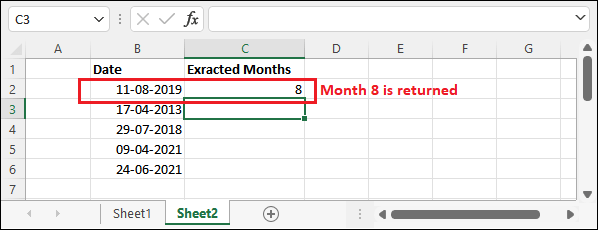It has returned month 8 after extracting it from B2 cell date (11-08-2021).

Step 4: Use the same formula to extract the month from other dates in this column or drag the resultant cell to use the same formula for other cells automatically.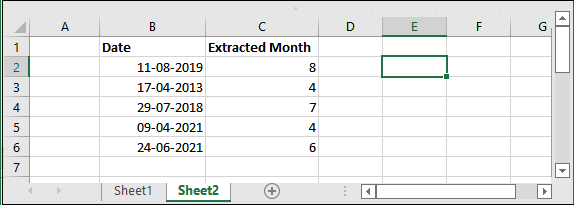See the extracted months for all dates in column C. It is one of the most easiest way to extract the date to month.

## Get the month name from date using TEXT function

Excel has another function (TEXT) to extract the month name from a date. The text function extracts the month and returns the month name from the date. This function accepts two parameters, i.e., date and type of value you want to convert. For example, mmmm for month name.

You will get the month name in different ways, such as – Jan or January. To get the month name as January, use mmmm and for month name as Jan, use mmm in second parameter of the TEXT function.

Use TEXT function as –

=TEXT(A2, m) to get the month number without zero, i.e., 3.

=TEXT(A2, mm) to get the month number with zero, i.e., 03.

=TEXT(A2, mmm) to get the month name in short, i.e., Sep.

=TEXT(A2, “mmmm”) to get the complete month name, i.e., September.

Here, suppose A2 contains a date on which we are performing these operations.

Example

Step 1: We take some sample data to see how to get the month name from a valid date by using the TEXT() function. The data for different dates is as follows:Step 2: Click on a blank cell where the extracted month name will appear and write the following formula for month extraction.

=TEXT(A2,”mmmm”)Step 3: It will here return the month name after extracting it from the date.Step 4: Use the same formula to extract the month from other dates in this column or drag the resultant cell to automatically use the same formula for other cells.See the extracted months for all dates in column B. This one is the best method to get the month name from a date.

## Convert the date to month using format cell

Format cell is select and click method of Excel. It is useful for users who are not good to use formulas in the Excel workbook. They can go for this method to convert the date to month. By using this method, you do not need to convert the date to month.

We will take the same dataset as used for the above methods so that you can compare both results.

Example

Step 1: It is the data of dates that we are using for this example.Step 2: Select one of the cells with date and press the Ctrl+1 shortcut key to open the Format Cell window panel.Step 3: Select the Custom under the Category options on the Number tab.You can also find this option as a dropdown list inside the Number section in the Home tab.

Step 4: Now, type mmmm inside the Type box and select it to get the full month names for the selected dates, and click OK.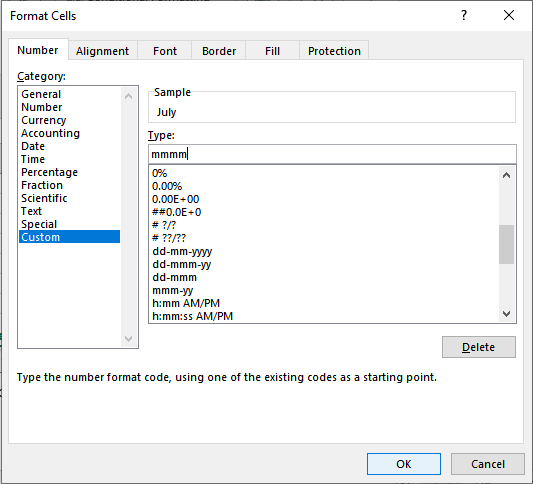Instead, you can also choose mmm for abbreviation of the month name.

Step 5: You can see that the month name is returned after extraction from the selected dates by using the format cell feature.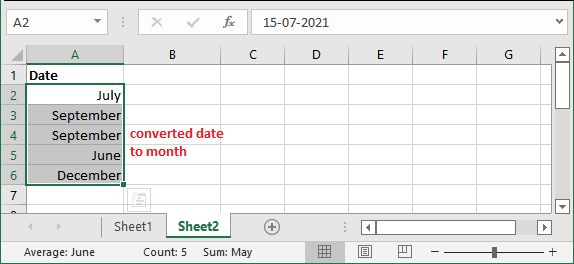You can convert and extract months for multiple dates at once.

## Date to month using SWITCH function

The SWITCH() is used with the MONTH() function to extract the month from a date. It returns the month name when you use this operation. However, the Excel users have to explicitly specify the month name for month number so that the month name can be extracted correctly without any interruption.

Syntax

The syntax for the SWITCH() with MONTH will be like as –

SWITCH(MONTH(A2), 1, “Jan”, 2, “Feb”, 3, “Mar”, 4, “Apr”, 5, “May”, 6, “June”, 7, “July”, 8, “Aug”, 9, “Sep”, 10, “Oct”, 11, “Nov”, 12, “Dec”)

This function will convert the month number to month name after extracting it from a date.

Example

Step 1: We have taken the following dates of data having month as a number. We will now use the SWITCH function to extract the month and convert it to month name.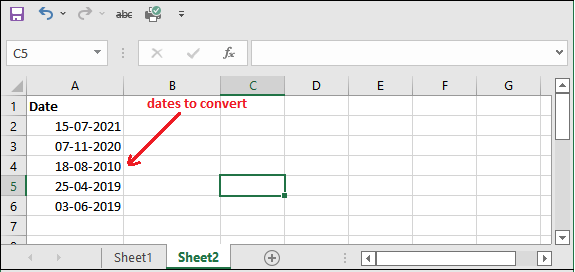Let’s see the below steps to learn how it will work.

Step 2: Now, we will use the formula that we explained above, i.e.,

=SWITCH(MONTH(A2), 1, “Jan”, 2, “Feb”, 3, “Mar”, 4, “Apr”, 5, “May”, 6, “June”, 7, “July”, 8, “Aug”, 9, “Sep”, 10, “Oct”, 11, “Nov”, 12, “Dec”)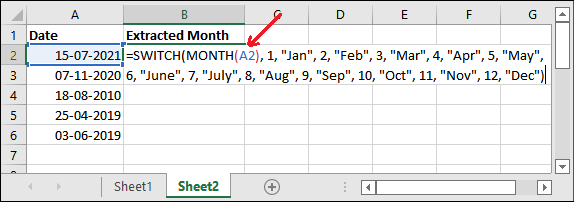Step 3: Hit the Enter key to get the result extracted from the date stored in the A2 cell.

See that it has returned July month for the respective date used in the formula.Similarly, apply the same formula on other cells to extract and convert the month name. See the result for all cells in the end.Excel has various methods to do this, you can select which you find most suitable for you.

## Convert the date to month using CHOOSE function

The CHOOSE() function is another way to convert the date to month by extracting the month from a date. If you find the above method difficult to use or does not meet your requirement, you can go for this method.

The CHOOSE() function converts a date to name of the month and day of the week. The users have to use the function accordingly.

`For example, to get the name of the month, use the CHOOSE() function as =CHOOSE(MONTH(A2), "Jan", "Feb", "Mar", "Apr", "May", "June", "July", "Aug", "Sep", "Oct", "Nov", "Dec").     In the same way, if you want the day of the week, use the function as =CHOOSE(WEEKDAY(A2), "Sun", "Mon", "Tue", "Wed", "Thu", "Fri", "Sat").   `

This method is more simpler than the SWITCH() function as you also need to explicitly specify the month number for each month.

Example

Step 1: It is the data of dates that we are using for this example to convert the date to month.Step 2: Now, we will use the formula that we explained above, i.e.,

=CHOOSE(MONTH(A2), “Jan”, “Feb”, “Mar”, “Apr”, “May”, “June”, “July”, “Aug”, “Sep”, “Oct”, “Nov”, “Dec”)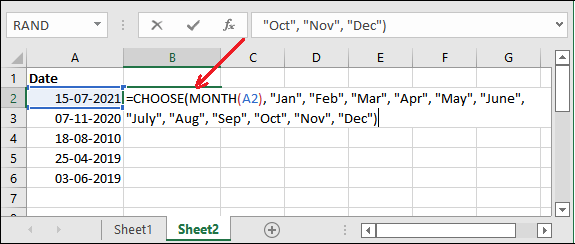Step 3: Hit the Enter key to get the result extracted from the date stored in the A2 cell.

See that it has returned July month for the respective date used in the formula.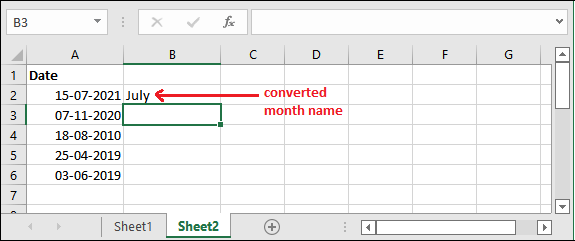Step 4: In the same way, if you want to know the weekday name, use the following formula.

=CHOOSE(WEEKDAY(A2), “Sun”, “Mon”, “Tue”, “Wed”, “Thu”, “Fri”, “Sat”)Step 5: Once again, hit the Enter key and get the result as weekday for the date stored in A3 cell.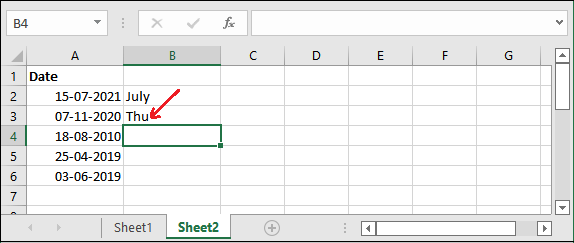Similarly, the Excel user can get the month from a date and besides this year, weekday as well. Excel provides various functions to get this.

Previous articleWhat is Macros
Next articleExcel Substring formula